﻿ 高校大学生分类管理中基于等价类的属性约简算法应用

# 高校大学生分类管理中基于等价类的属性约简算法应用Application of Attribute Reduction Algorithm Based on Equivalent Class in Classification Management of College Students

Abstract: Based on the particularity of the college student group, this paper proposes to use formal concept analysis for classification management of students, which provides valuable reference for student workers. This paper applies characteristics of attribute and equivalent class to attribute reduction, puts forward judging theorems of attribute reduction with demonstration; secondly, proposes an algorithm of attribute reduction and output, applies this algorithm to classification management of college students for the first time. Specifications are as follows: first of all, establish a binary tree with attributes and obtain sequences of PreOrder, InOrder and PostOrder; delete an attribute; then perform intersection between the rest of attributes and each intent of concepts gradually, if each result contains single element, then this attribute can be removed, otherwise, skip this attribute, repeat the procedures above, consistent set can be obtained, reduction set which involves the minimum elements of consistent set can be output as well; subsequently, discusses the time complexity, this paper reduces the time complexity of reduction set output to polynomial level for the first time. Comparing with other algorithms on runtime and ability of classification, experi-mental results show that the proposed method approves feasibility and accuracy, in the end, draws a conclusion and discusses open issues.

1. 引言

1966年，克拉克(Clark)和特罗(Trow)从大学认同度(identification with college)和参与思想(involvement with ideas)两个维度将学生分为4类，分别为：学术型(academic)、社交型(collegiate)、职业型(vocational)、不墨守成规型(nonconformist)；李志峰  把学生分为高度匹配型、独立型、被动顺应型和排斥型等几种类型，按照学生的不同类型形成多样化和更为自主性的管理范式，有利于调动学生学习积极性，促进学生成长成才成功。国内研究大部分是以学生成绩、学习行为等依据进行分类，这种分类关注的是学习结果，忽视了其他影响因素。学生分类管理，不能简单以学习成绩为依据，学习成绩好并不一定思想行为就没有问题，分类管理的意义在于引导学生认识到一个真正优秀的人应该尽可能各方面都变得优秀。这才是正确的教育过程。

2. 概念格理论基础

${A}^{\ast }=\left\{y|\forall x\in A,I\left(x,y\right)=1\right\}$

${B}^{\ast }=\left\{x|\forall y\in B,I\left(x,y\right)=1\right\}$

${A}^{\ast }$ 是对象集A共同具有的属性集， ${B}^{\ast }$ 是共同具有属性集B的对象集。 $〈A,B〉$ 是一个概念当且仅当 $A={B}^{*}$$B={A}^{*}$ 。其中A为概念的外延，B为概念的内涵。

$B\subseteq {B}_{1}$ 并且没有 $〈{A}_{2},{B}_{2}〉$ 满足 $B\subseteq {B}_{2}\subseteq {B}_{1}$ ，则 $〈{A}_{1},{B}_{1}〉$$〈A,B〉$ 的后继。

3. 属性约简相关理论

$L\left({I}_{1}\right)$ 细于 $L\left(I\right)$$L\left(I\right)$ 细于 $L\left({I}_{1}\right)$ ，则 $L\left({I}_{1}\right)$$L\left(I\right)$ 同构，记为 $L\left({I}_{1}\right)\cong L\left(I\right)$

(1) 核心属性： $C=\cap {E}_{i}$ ，其属于每个约简。

(2) 相对必要属性： $J=\cup {E}_{i}-\cap {E}_{i}$ ，其属于某些约简但不属于每个约简。

(3) 不必要属性： $K=Y-\cup {E}_{i}$ ，其不属于任一约简。

4. 属性约简算法

(1) 根据属性构造二叉树，调用二叉树前序、中序、后序算法，得到前序、中序、后序属性序列。

(2) 生成条件概念：

(3) 将核心属性，存入数组 $Core\left[|Y|\right]$

for each $C\left[i\right]$

if( $C\left[i\right].no==1$ ) $i\to Core\left[|Y|\right]$ ;

(4) 等价类法进行属性约简，根据前序、中序、后序序列，调用以下算法三次：

{E=Y;

for (i=1; $i\le |Y|$ ; i++)/*从第i个属性开始，依次判断属性 $Y\left[i\right]$ 是否可删*/

{if ( $Y\left[i\right]$ in $Core\left[|Y|\right]$ ) continue;/*核心属性不能约*/

$E=E-Y\left[i\right]$ ;/*将去掉属性 $Y\left[i\right]$ 后的剩余属性存放于E中*/

for each $C\left[j\right]$

{k=0;

$s\to data=E\cap C\left[j\right].I\left[|Y|\right]$ ;/*将剩余属性E与每个概念的内涵求交存入节点S的数据域，k为计数器，记录相交属性的个数*/

$s\to next=p\left[k\right]\to next$

$p\left[k\right]\to next=s$ ;}/* $p\left[k\right]$ 为指针指示相交后有k个属性的单链表，采用头插法插入每个结点*/

k=0;

while( $p\left[k\right]\to next$ )/*比较各非空的单链表*/

{ $q=p\left[k\right]\to next$ ;

p=q;

while( $q\to next$ )

{ $p=p\to next$ ;

while(p)

if( $p\to data==q\to data$ ) break;/*两个结点的数据域相等，说明该等价类不是单元素集，则剩余结点无需比较*/

else $p=p\to next$ ;

if(p!=NULL) break;

$q=q\to next$ ;

p=q;

}

if( $q\to next$ ) break;

k++;

}

if( $k ) $Y\left[i\right]\to E$ ;/*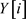不能约，存入数组E*/

}

5. 实验分析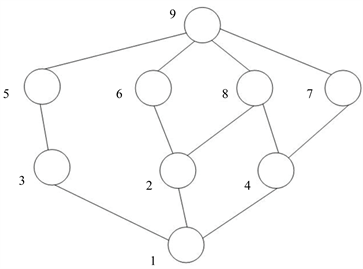Figure 1. The concept lattice

(1)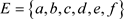(2)，删a，因为a不是核心属性，所以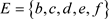(3) 依次求交集，得到各单链表：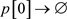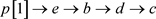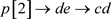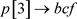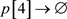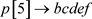(4) 各单链表中无相同元素，所以开始删b，因为b不是核心属性，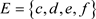(5) 依次求交集，得到各单链表：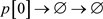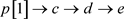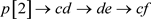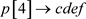(6)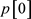中存在相同元素，所以b不能删，(7) 因为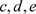是核心属性，所以结束本次循环；

(8) 因为f不是核心属性，所以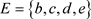(9) 依次求交集，得到各单链表：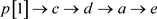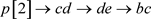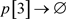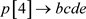(10) 各单链表中无相同元素，最终输出约简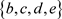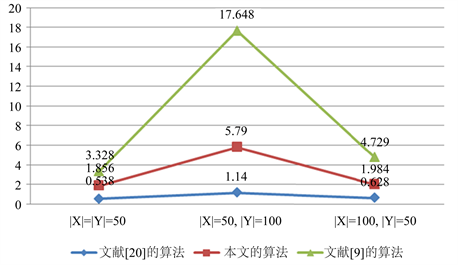Figure 2. The efficiencyTable 3. Formal context after reductionTable 4. Concepts after reductionTable 5. Comparison with ability of classification

6. 结束语

NOTES

*通讯作者。

 李志峰, 罗梦辉, 王春春. 基于学生分类发展的本科教学管理制度变革——以“自我-社会认识”为分析视角[J]. 国家教育行政学院学报, 2016(12): 51-56+65.

 李珍, 刁钢, 赵慧峰. 基于大数据分析的学生学业分类管理体系——河北农业大学商学院新生入学成绩的K-Mean聚类分析[J]. 河北农业大学学报(农林教育版), 2018, 20(5): 96-99.

 Wille, R. (1982) Restructuring Lattice Theory: An Approach Based on Hierarchies of Concepts. In: Rival, Ordered Sets, Reidel, Dordrecht, 445-470.
https://doi.org/10.1007/978-94-009-7798-3_15

 马垣, 马文胜. 概念格多属性渐减式构造[J]. 软件学报, 2015, 26(12): 3162-3173.

 李进金, 张燕兰, 吴伟志, 等. 形式背景与协调决策形式背景属性约简与概念格生成[J]. 计算机学报, 2014, 37(8): 1768-1772.

 Skowron, A. and Rauszer, C. (1992) The Discernibility Matrices and Functions in Information Systems. In: Decision Support, Handbook of Applications and Advances of the Rough Sets Theory, Springer, Dordrecht, 331-340.
https://doi.org/10.1007/978-94-015-7975-9_21

 Sabita Mahapatra, S., et al. (2010) Attribute Selection in Marketing: A Rough Set Approach. IIMB Management Review, 22, 6-24.
https://doi.org/10.1016/j.iimb.2010.03.001

 王霞, 张文修. 概念格的属性约简与属性特征[J]. 计算机工程与应用, 2008, 44(12): 1-4.

 王璨. 基于模糊形式背景的概念格属性约简算法研究[J]. 电子设计工程, 2016, 24(10): 17-20.

 莫京兰, 翁世洲, 李金海. 一种基于属性划分的序信息系统并行约简算法[J]. 工程数学学报, 2014, 31(5): 633-641.

 徐怡, 王泉, 霍思林. 粒计算中基于属性分类的形式概念属性约简[J]. 控制与决策, 2018, 33(12): 2203-2207.

 Jia, X.Y., Rao, Y., Shang, L. and Li, T.J. (2019) Similarity-Based Attribute Reduction in Rough Set Theory: A Clustering Perspective. International Journal of Machine Learning and Cybernetics, 2019, 1-14.
https://doi.org/10.1007/s13042-019-00959-w

 Wang, C., Yu, X., Xue, C.M., et al. (2013) Research on Com-puting the Covers of a Given Concept. Applied Mechanics and Materials, 467, 496-500.
https://doi.org/10.4028/www.scientific.net/AMM.467.496

 Wang, C. and Yu, X. (2014) Research on Attribute Reduction Based on L-Context. 2014 11th International Conference on Fuzzy Systems and Knowledge Discovery (FSKD), Xiamen, 19-21 August 2014, 450-455.
https://doi.org/10.1109/FSKD.2014.6980875

 Wang, C. (2016) Research on Algorithm of Attribute Reduction Based on Concept with Introducer. 2016 12th International Conference on Natural Computation, Fuzzy Systems and Knowledge Discovery (ICNC-FSKD 2016), Changsha, 13-15 August 2016, 1318-1323.
https://doi.org/10.1109/FSKD.2016.7603369

 Wang, C. (2017) Attribute Reduction Based on Object Concepts. 2017 13th International Conference on Natural Computation, Fuzzy Systems and Knowledge Discovery (ICNC-FSKD 2017), Guilin, China, 29-31 July 2017, 1802-1806.
https://doi.org/10.1109/FSKD.2017.8393040

 王璨, 侯洪凤, 郭文书, 等. 基于决策规则的属性约简算法研究[J]. 山西大学学报(自然科学版), 2016, 39(3): 349-356.

 张文修, 魏玲, 祁建军. 概念格的属性约简理论与方法[J]. 中国科学E辑信息科学, 2005, 35(6): 628-639.

 宫玺. 概念格的构造、约简及形式概念分析的应用[D]: [硕士学位论文]. 辽宁: 辽宁科技大学, 2008: 25-34.

 张文修, 仇国芳. 基于粗糙集的不确定决策[M]. 北京: 清华大学出版社, 2005: 75-100.

Top